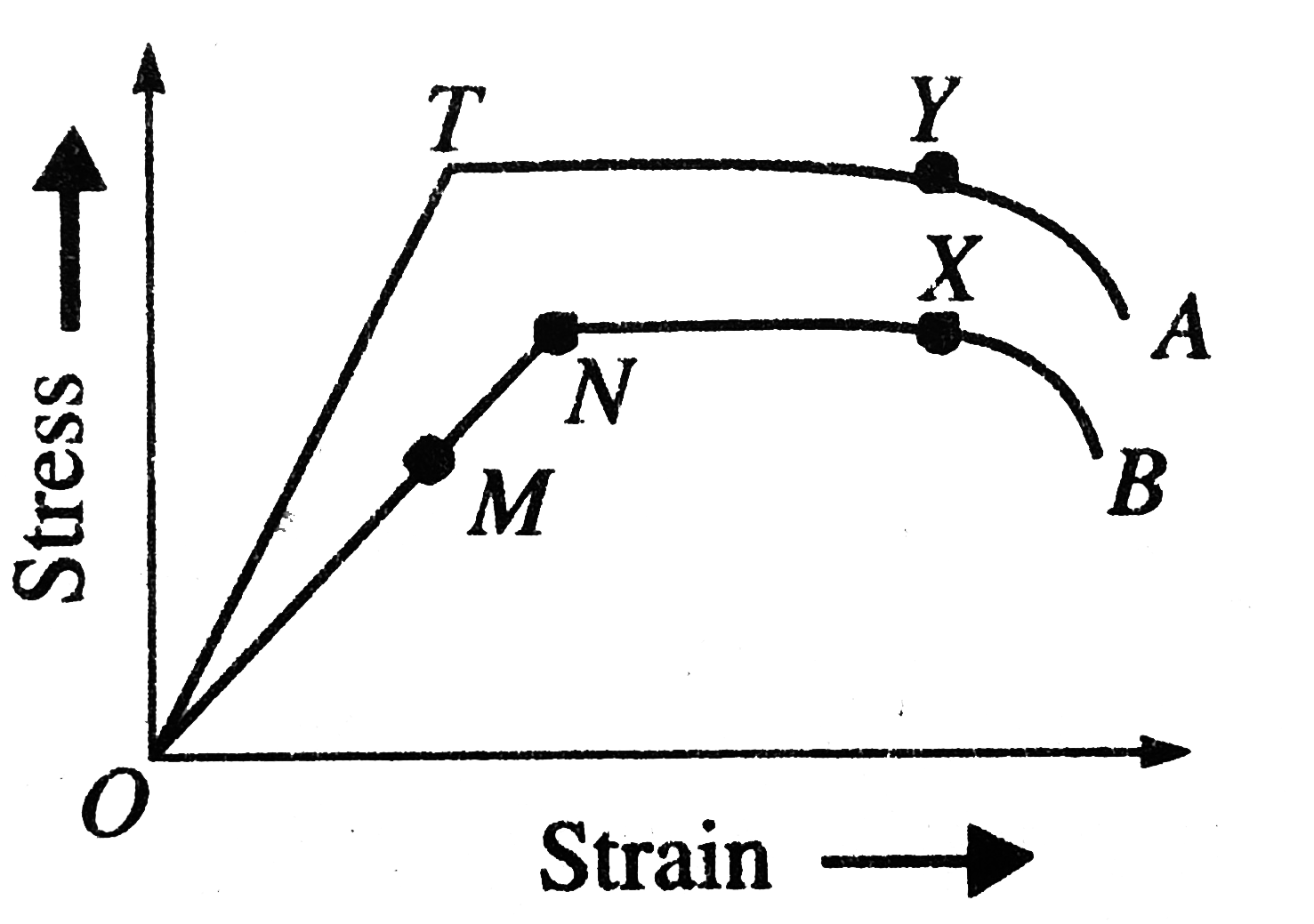# Materials get deformed when force is applied. Some of them regain their status when the applied force is removed. They are termed as elastic. Those of

31 views
in Physics
closed
Materials get deformed when force is applied. Some of them regain their status when the applied force is removed. They are termed as elastic. Those of which not regaining are called plastic. There may be delay in the regaining in some materials. They are said to have got elastic aftereffect, since they have gone beyond the elastic limit. Repeated application and removal of force leads to fatigueness in the material. Fatigued materials may break at any point time and so are avoided.
The stress strain graph for two materials A and B is shown in the following figure:The strength of the material A and B is S_(A) and S_(B), respectively, while the longevity of plastic behaviour is L_(A) and L_(B). Then
A. S_(A)gtS_(B),L_(A)ltL_(B)
B. S_(A)=S_(B),L_(A)=L_(B)
C. S_(A)gtS_(B),L_(A)gtL_(B)
D. S_(A)ltS_(B),L_(A)ltL_(B)

by (87.1k points)
selected

TY and NX are showing the plastic region in A and B.
so, L_(A)gtL_(B)
Since A withstands more stress before yielding than B, S_(A)gtS_(B)
so c is correct and the rest are incorrect.